# Concept: Triangles

INTRODUCTION

Triangles are closed figures containing three angles and three sides.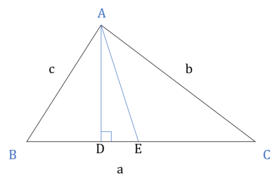​​​​​​​

AD here is the altitude from vertex A. AE is the median.

GENERAL PROPERTIES

• Side opposite to largest angle is largest and side opposite to smallest angle is the smallest.

⇒ If ∠A > ∠B > ∠C, then a > b > c.
• The sum of all three angles of a triangle is 180°.

⇒ ∠A + ∠B + ∠C = 180°
• The exterior angle is equal to sum of the two opposite interior angles A and B, i.e. α = ∠A + ∠B

•​​​​​​​

• Sum of all three exterior angles of a triangle is 360°.

• The sum of two sides of a triangles is greater than the third side.

a + b > c
b + c > a
c + a > b
• The difference of any two sides of a triangle is less than the third side.

|a - b| < c
|b - c| < a
|c - a| < b
• Perimeter of triangle = a + b + c

• Semi perimeter (s) = $\frac{\left(a + b + c\right)}{2}$

AREA OF A TRIANGLE

• Area of a triangle = $\frac{1}{2}$ × base × height = $\frac{1}{2}$bh

•​​​​​​​

• Area of a triangle = $\frac{1}{2}$abSinC = $\frac{1}{2}$bcSinA = $\frac{1}{2}$acSinB

• Area of a triangle = $\sqrt{s\left(s-a\right)\left(s-b\right)\left(s-c\right)}$

• Area of a triangle = r × s. [r = inradius]

• Area of a triangle = $\frac{abc}{4R}$. [R = circumradius]

• Triangles on same set of parallel lines and having same base are of equal area. [Since their base is equal and their height is also same.]

SIN and COSINE RULES

SIN RULE

$\frac{a}{SinA}$ = $\frac{b}{SinB}$ = $\frac{c}{SinC}$

COSINE RULE

• CosA = $\frac{{b}^{2}+{c}^{2}-{a}^{2}}{2bc}$

⇒ a2 = b2 + c2 – 2bcCosA
• CosB = $\frac{{c}^{2}+{a}^{2}-{b}^{2}}{2ca}$

⇒ b2 = c2 + a2 – 2caCosB
• CosC = $\frac{{a}^{2}+{b}^{2}-{c}^{2}}{2ab}$

⇒ c2 = a2 + b2 – 2abCosC

TYPES OF TRIANGLES

Based on Angles

Acute Triangle​​​​​​​

• All angles are < 90°

• All general properties of ∆s apply.

• AC2 = AB2 + BC2 – 2 × BC × BD (D is on BC such that AD ⊥ BC)

• Check for an Acute triangle.

In a ∆, if c is the longest side and c2 < a2 + b2, then it is an Acute triangle.

Right Triangle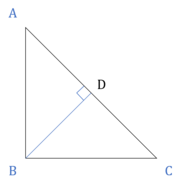​​​​​​​

• Exactly one angle is 90°.

• All general properties of ∆s apply.

• Hypotenuse2 = Base2 + Perpendicular2

• Area = $\frac{1}{2}$ × AB × BC

• Altitude BD = $\frac{\left(AB × BC\right)}{AC}$

• Altitude BD = $\sqrt{\mathrm{AD}×\mathrm{CD}}$

• Midpoint of the hypotenuse is equidistant from the three vertices i.e. EA = EB = EC. It implies that the circumradius of a right-angled triangle is half of the hypotenuse.

⇒ R = $\frac{Hypotenuese \left(c\right)}{2}$
• Inradius = $\frac{\left(a + b - c\right)}{2}$

• Check for a Right triangle.

In a ∆, if c is the longest side and c2 = a2 + b2, then it is a Right triangle.

Obtuse Triangle​​​​​​​

• Exactly one angle is > 90°.

• All general properties of ∆s apply.

• AC2 = AB2 + BC2 + 2 × BC × BD (D is on BC such that AD ⊥ BC)

• In a obtuse triangle, circumcenter and the orthocenter lie outside the triangle.

• Check for an Obtuse triangle.

In a ∆, if c is the longest side and c2 > a2 + b2, then it is an Obtuse triangle.

Based on Sides

Scalene Triangle

• No 2 sides are equal.

• No 2 angles are equal.

• All the general properties of a triangle apply.

Isosceles Triangle​​​​​​​

• Exactly 2 sides are equal.

• The angles opposite to the equal sides are also equal.

• All the general properties of a triangle apply.

• Altitude from the vertex containing equal sides is the Median as well the Angle Bisector (i.e. it divides the in ∆ two equal halves).

• In an isosceles triangle, all the four points, viz, circumcenter, incenter, centroid and orthocenter lie on the median drawn from the vertex contained by equal sides to the non-equal side.

Equilateral Triangle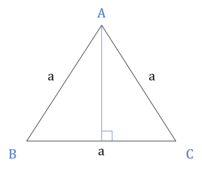​​​​​​​

• All sides are equal (a).

• All angles are equal to 60°.

• All the general properties of a triangle apply.

• Height of the triangle = $\frac{\sqrt{3}}{2}$a

• Area of the triangle = $\frac{\sqrt{3}}{4}$a2

• Inradius = $\frac{a}{2\sqrt{3}}$

• Circumradius = $\frac{a}{\sqrt{3}}$

• Altitudes, Medians, Angle Bisectors are all same.

• In an equilateral triangle all the four points viz, circumcenter, incenter, centroid and orthocenter coincide.

• Equilateral triangle has the maximum area, out of all the triangles that can be inscribed in a given circle.

Triangles to Remember

30° – 60° – 90° triangle​​​​​​​

• Side opposite to 30° = half of hypotenuse.

• Side opposite to 60° = $\frac{\sqrt{3}}{2}$ × hypotenuse

45° – 45° – 90° triangle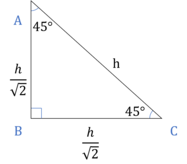​​​​​​​

• Each of the smaller sides = $\frac{1}{\sqrt{2}}$ × hypotenuse.

• Hypotenuese = √2 × side

SIMILARITY OF TRIANGLES

If two triangles ABC and PQR are similar, then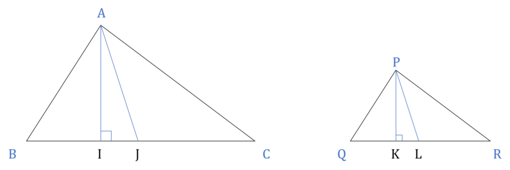​​​​​​​

• Corresponding angles are equal i.e., ∠A = ∠P ; ∠B = ∠Q ; ∠C = ∠R

• Ratio of corresponding sides are equal

i.e., $\frac{AB}{PQ}$ = $\frac{BC}{QR}$ = $\frac{CA}{RP}$
• Ratio of corresponding altitudes / medians / perimeter / circumradius / inradius = Ratio of corresponding sides

i.e., $\frac{AB}{PQ}$ = $\frac{BC}{QR}$ = $\frac{CA}{RP}$ = $\frac{AI}{PK}$ (altitude) = $\frac{AJ}{PL}$ (median)
• Ratio of areas = Square of ratio of sides

i.e.,  = ${\left(\frac{AB}{PQ}\right)}^{2}$

Corresponding sides are those sides in both triangles which are opposite the equal angle in both triangles.

Proof of Similarity
• AAA Rule:

i.e., If all corresponding angles are equal, then the two triangles are similar.
•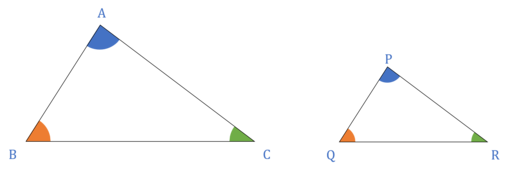​​​​​​​

Here, ∠A = ∠P, ∠B = ∠Q and ∠C = ∠R. Hence, triangles ABC and PQR are similar triangles.

• SAS (ratio) Rule:

i.e., If an angle is equal and corresponding sides containing the angle are in same proportion.
•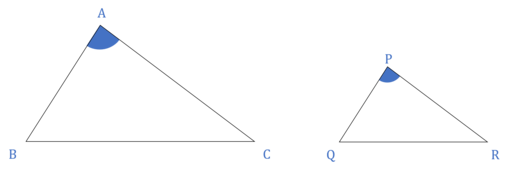​​​​​​​

Here, $\frac{AB}{PQ}$ = $\frac{AC}{PR}$ and ∠A = ∠P. Hence, triangles ABC and PQR are similar triangles.

• SSS (ratio) Rule:

i.e., If the three corresponding sides are in same proportion.
•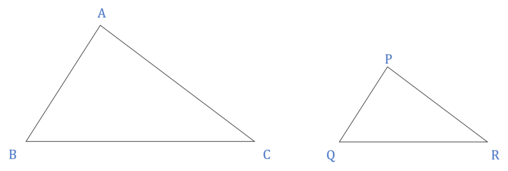​​​​​​​

Here, $\frac{AB}{PQ}$ = $\frac{BC}{QR}$ = $\frac{CA}{RP}$. Hence, triangles ABC and PQR are similar triangles.

• RHS (ratio) Rule:

i.e., If the hypotenuse and one of the smaller sides are in same proportion of two right triangles.
•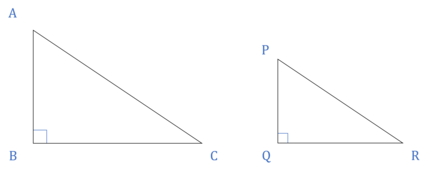​​​​​​​

Here, $\frac{AB}{PQ}$ = $\frac{AC}{PR}$ and ∠B = ∠Q = 90°. Hence, triangles ABC and PQR are similar triangles.

All equilateral triangles are similar to each other. [AAA Rule]

Special case of a Right triangle

In a right triangle if we draw an altitude from the right vertex, we get 2 smaller right triangles which are similar to each other and also similar to the original right triangle.SOME IMPORTANT RESULTS
• Here, ∆ABC ~ ∆ADB ~ ∆BDC
• BD2 = AD × CD
• AB2 = AD × AC
• BC2 = CD × CA
• AB × BC = BD × AC

CONGRUENCY OF TRIANGLES

All corresponding sides and corresponding angles are equal i.e., when two triangles are exactly same, they are called congruent triangles.

Proof of Congruency
• SSS Rule:

i.e., If the three corresponding sides are equal, then the two triangles are congruent.
•​​​​​​​

Here, AB = PQ, BC = QR and AC = PR. Hence the two triangles are congruent triangles.

• SAS Rule:

i.e., If the two corresponding sides are equal and the contained angle is also equal.
•​​​​​​​

Here, AB = PQ, AC = PR and ∠A = ∠P. Hence the two triangles are congruent triangles.

• AAS / ASA / SAA Rule:

i.e., Two angles are equal and a side is equal.
•​​​​​​​

Here, AB = PQ, ∠A = ∠P and ∠B = ∠Q. Hence the two triangles are congruent triangles.

• RHS Rule:

i.e., In two right triangles, hypotenuses are equal and one of the sides are equal.
•​​​​​​​

Here, AB = PQ, AC = PR and ∠B = ∠Q = 90°. Hence the two triangles are congruent triangles.

GEOMETRIC CENTERS

Centroid (Medians)

Centroid is the meeting point of the three medians of a triangle.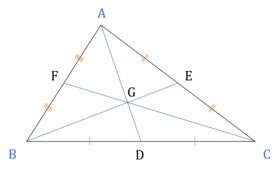​​​​​​​

• The median divides the triangle into two equal areas. In the figure, area ∆ABD = area ∆ACD = area ∆BCE = area ∆BAC = area ∆CBF = area ∆CAF = ½ (area ∆ABC).

• The centroid divides a median in the ratio 2 : 1. In the figure $\frac{AO}{OD}$ = $\frac{BO}{OE}$ = $\frac{CO}{OF}$

• The three medians divide a triangle in 6 triangles of equal areas.

i.e., area ∆GBD = area ∆GCD = area ∆GCE = area ∆GAE = area ∆GAF = area ∆GBF = ⅙ (area ∆ABC)
• Median drawn to longer side is shorter in length.

• Medians drawn to equal sides are equal in length.

• Sum of two medians is greater than the third median.

• Difference of two medians is less than the third median.

Apollonius Theorem

• AB2 + AC2 = 2(AD2 + BD2)
• BC2 + BA2 = 2(BE2 + CE2)
• CB2 + CA2 = 2(CF2 + BF2)

• 3(AB2 + BC2 + AC2) = 4(AD2 + BE2 + AF2)

LINE JOINING THE MIDPOINTS​​​​​​​

• The line joining the mid-points of any two sides of a triangle is parallel to the third side and half of it.

⇒ FE ∥ BC, DE ∥ AB, DF ∥ AC
⇒ FE = BC/2, DE = AB/2, FD = AC/2
• If we join the midpoints of three sides of a triangle, we get 4 triangles of equal areas.

i.e., area ∆DEF = area ∆AFE = area ∆BDF = area ∆DEC = ¼ (area ∆ABC)

Corollary: If a line parallel to the base and passes through the midpoint of one side, it will pass through the midpoint of the other side also.

Orthocenter (Altitudes)

Othocenter is the meeting point of the three altitudes of a triangle.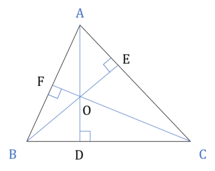​​​​​​​

• ∠AOC and ∠B are supplementary angles.

• AD + BE + CF < AB + BC + CA

• In a right-angled triangle, the vertex containing the right angle is the orthocenter.

Incenter (Angle Bisectors)

If we draw angle bisectors of all three angles of a triangle, they all meet at a single point called Incenter.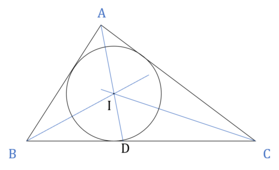​​​​​​​

• Incenter is equidistant from all 3 sides.

• ∠BIC = 90 + $\frac{A}{2}$

• Area of triangle = r × s

• Inradius of a right-angled ∆ = (P + B - H)/2

Angle Bisector Theorem

In a triangle if AD is the anlge bisector of ∠A meeting BC at D, then

$\frac{AB}{AC}$ = $\frac{BD}{CD}$

Circumcenter (Penpendicular Bisectors)

If we draw perpendicular bisectors of all three sides of a triangle, they all meet at a single point called Circumcenter.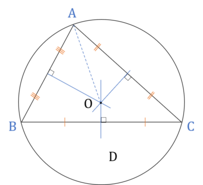​​​​​​​

• Circumcenter is equidistant from all 3 vertices.

• Area of triangle = $\frac{abc}{4R}$

• ∠BOC = 2∠BAC

• Inradius of a right-angled ∆ = (P + B - H)/2

• Circumradius of a right-angled ∆ = H/2

Special Case of an Equilateral triangle

• In an equilateral ∆, all four geometric centers coincide.
• Height / Median of the triangle = $\frac{\sqrt{3}}{2}$a
• Inradius = $\frac{a}{2\sqrt{3}}$
• Circumradius = $\frac{a}{\sqrt{3}}$

Special Case of a Right triangle

• In a right ∆, the vertex with right angle is the orthocenter.
• In a right ∆, midpoint of the hypotenuse is equidistant from the three vertices i.e. DA = DB = DC. It implies that the circumradius of a right-angled triangle is half of the hypotenuse.
• Inradius = (P + B - H)/2

SOME IMPORTANT RESULTS
• Centroid and Incenter will always lie inside the triangle.
• For an acute angled triangle, the Circumcenter and the Orthocenter will lie inside the triangle.
• For an obtuse angled triangle, the Circumcenter and the Orthocenter will lie outside the triangle.
• For a right angled triangle the Circumcenter will lie at the midpoint of the hypotenuse and the Orthocenter will lie at the vertex at which the angle is 90°.
• The orthocenter, centroid, and circumcenter always lie on the same line known as Euler Line. The orthocenter is twice as far from the centroid as the circumcenter is.
• In an isosceles triangle, all the four points, viz, circumcenter, incenter, centroid and orthocenter lie on the median drawn from the vertex contained by equal sides to the non-equal side.
• If the triangle is equilateral, all four are the same point.
• Inradius is less than half of the smallest altitude.

Ex-Circle

• Excircle is formed by intersection of 1 internal ∠bisector and 2 external ∠bisectors

• Exradius (rA)) = $\frac{\mathrm{Area}}{\sqrt{\left(s - a\right)}}$

Basic Proportionality Theorem

A line drawn parallel to one of the three sides of a triangle divides the other 2 sides in same proportion.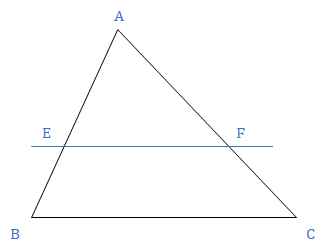​​​​​​​

Conversely, If E and F are two points dividing AB and AC in same proportion, then EF will be parallel to BC.

Here, triangle AEF is similar to triangle ABC.

Line joining the mid-points of two sides is parallel to third side.

## Feedback

Help us build a Free and Comprehensive Preparation portal for various competitive exams by providing us your valuable feedback about Apti4All and how it can be improved.